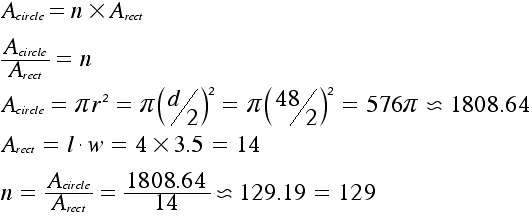SEARCH HOMEMath Central Quandaries & QueriesQuestion from Susan, a parent: How many 4" X 3.5" wide escort cards will fit on a 48" diameter table. These are for my daughters wedding. I have already added the extra spacing between the cards into the size of the cards.Thanks for your helpHi Susan,

To be able to find how many cards will fit on the table, we need to find the area of the table and each card. The number of cards times the area of each card will approximately equal the area of the circular table. To find the maximum number of cards (which I will represent with "n") that will fit on the table, we need to isolate the n we can divide both sides by the area of each card. As you can see below, the number of cards is a ratio of the area of the table and the area of each card.Remember that the number of cards you use is always a whole number so you ALWAYS round down to the nearest whole number. This is the maximim number of cards possible. Since the table is round the spacing between the cards will not be uniform and the precise number will depend on the placement of the cards.

Janice and HarleyMath Central is supported by the University of Regina and The Pacific Institute for the Mathematical Sciences.